Next: About this document ... Up: lab_template Previous: lab_template

Subsections

# Solving Equations with Maple

## Introduction

The purpose of this lab is to find solutions to one equation.

## Solving a function or an expression algebraically

You can set an expression or function equal to another expression, function, or number inside a solve command.As an example, you may want to find where the following two parabolas intersect.
> g := 9*x^2-14;
> h:=-x^2;
> plot([g,h],x=-2..2);
> solve(g=h,x);

The plot shows that there are two intersection points and the solve command finds both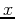values.It is good to get into the habit of naming your output so you can use it in a later command. Giving thevalues a name makes it easy to plug them into the function to find thevalues.
> ip:=solve(g=h,x);

Since there are twovalues called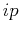, use [ ] to call up the one you want.
> eval(g,x=ip);
> eval(h,x=ip);

Therefore the two intersection points are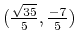and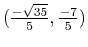. This seems like the answer shown on the graph.

## Solving a function or an expression numerically

If you would like to find where the following function crosses the horizontal line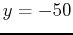you can try the solve command.
> j:=x->2*x^3-15*x^2-2*x+5;
> k:=x->-50;
> plot([j(x),k(x)],x=-3..8);

The graph shows there should be three answers.
> solve(j(x)=k(x),x);

That is some scary output! So instead of using the algebraic solve try the numerical fsolve.
> fsolve(j(x)=k(x),x);


## Some more strange output

If you want to find where the following function crosses the x-axis, just set it equal to zero.
> f:=theta->-1/2*theta+sin(theta);
> plot(f(theta),theta=-8*Pi..8*Pi);
> solve(f(theta)=0,theta);

Wow, what is that?!?! We know from the graph that there should be three answers and solve wasn't a great option so try fsolve again.
> fsolve(f(theta)=0,theta);

Where are the other two answers!? This is actually how fsolve usually works. It shoots for one answer and only gives that one. But you can tell fsolve where to look by getting an idea from the graph and typing that domain into the fsolve command.
> a:=fsolve(f(theta)=0,theta=-5..-1);
> b:=fsolve(f(theta)=0,theta=-1..1);
> c:=fsolve(f(theta)=0,theta=1..5);

To find thevalues just plug in the names of thevalues.
> f(a);
> f(b);
> f(c);

(Of course the y-values are zero!)

## Exercises

1. Given the expression,
A)
Plot the expression and in text state how many roots it has. That is, how many times the it crosses the x-axis.(Experiment with domain values until you find values that show the crossing points clearly.)
B)
Compare outputs from the Maple solve and fsolve command to find thevalues of where it crosses the x-axis. State the real roots in text.
C)
Use the Maple eval command to evaluate the expression at each of the roots to show that they are zero.
2. Given the functions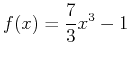and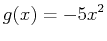A)
Plot the functions. Again experiment with domain values until the intersection points are clear. Then state in text how many intersection points you see.
B)
Compare outputs from the Maple solve and fsolve command find the intersection points. Label thevalues with a variable in front of the solve or fsolve command.
C)
Find all correspondingvalues and state the intersection points in text. (When writing your text sentence use 3 significant figures for the answer. Be sure to round properly!)
3. Given the functions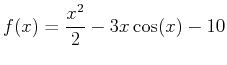andA)
Plot the functions. Again experiment with domain values until the intersection points are clear. Then state in text how many intersection points you see.
B)
Using the solve command find the intersection points. Label thevalues with a variable in front of the solve command. How manyvalues does the solve command find? Use the fsolve command to find the rest of the intersection poins. Label thevalues by giving each fsolve command a name.
D)
Find all correspondingvalues and state the intersection points in text.Next: About this document ... Up: lab_template Previous: lab_template
Dina J. Solitro-Rassias
2015-09-10